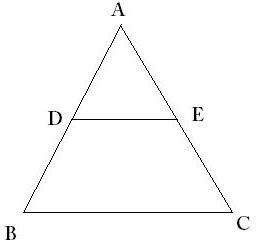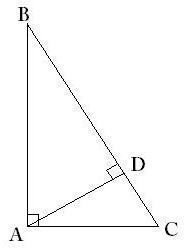# Tips for Teaching the Properties of Similar Triangles

Page content

## What are Similar Triangles?

A triangle is a planar closed figure with three sides, i.e, a three sided polygon. The basic elements of a triangle are its three sides and the three angles they form.

As a general rule, two objects are called ‘similar’ if they have the same shape or one is the enlargement of another. Two triangles are called ‘similar’ if all three of their angles match. However, the sides of these triangles can be different. If the corresponding sides also match, along with the angles, the triangles are called ‘congruent’. If two triangles are congruent to each other, they are also similar but the converse is not true. Mathematically, similarity is represented by the symbol “~” . E.g, ABC ~ PQR (read as triangle ABC is similar to triangle PQR).

Left Bottom: A pair of similar triangles.

## Properties of Similar Triangles

1. The corresponding angles are equal. E.g, if PQR ~ ABC, then

angle P = angle A

angle Q = angle B

angle R = angle C

2. The corresponding sides are in the same proportion. E.g, if PQR ~ ABC, then

PQ/AB = QR/BC = PR/AC

3. If two triangles are similar, they remain similar even after rotation or reflection about any axis as these two operations do not alter the shape of the triangle.

## Proving Similarity

Three main approaches are used to prove similarity of two triangles. We will use triangles ABC and PQR in examples in this section.

1. AA :

Any two pairs of angles of the two triangles are the same. E.g,

angle A = angle P

and angle B = angle Q

=> ABC ~ PQR

As the sum of the angles of any triangle is a constant, angle C = angle R.

2. SSS :

The corresponding sides of the triangles are in proportion. E.g,

AB/PQ = AC/PR = BC/QR

=> ABC ~ PQR

3. SAS :

Any one of the corresponding angles of the two triangles is equal and the sides containing it are in proportion. E.g,

angle A = angle P

and AB/PQ = AC/PR

=> ABC ~ PQR

## Solved Examples1. In the figure to the left, D and E are mid-points of AB and AC respectively. Prove that ABC ~ ADE.

Solution: We will use the SAS method of proving similarity in this case:

angle A = angle A (common angle)

AD/AB = AE/AC = 1/2 (D and E are mid-points of AB and AC)

Hence, proved.

2. In the figure below (left), BAC is a right angled triangle with a right angle at A. AD is the perpendicular from vertex A to side BC.Solution: In right triangle BAC, angle B = 90o - angle C … (1)

In right triangle CDA, angle CAD = 90o - angle C … (2)

By (1) and (2),